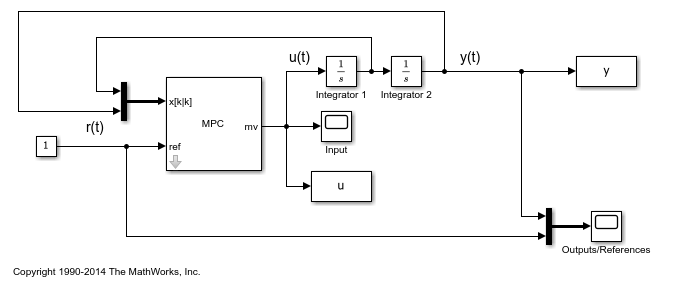Documentation

Custom State Estimation

Model Predictive Control Toolbox™ software allows you to override the default controller state estimation method.

To do so, you can use the following methods:

• You can override the default Kalman gains,and, using the setEstimator function. To obtain the default values from the controller use getEstimator. These commands assume that the columns ofandare in the engineering units of the measured plant outputs. Internally, the software converts them to dimensionless form.

• You can use the custom estimation option, which skips all Kalman gain calculations within the controller. When the controller operates, at each control interval you must use an external procedure to estimate the controller states and provide these state estimates to the controller.

Custom state estimation is not supported in MPC Designer.

Consider the case of a double integrator plant for which all of the plant states are measurable. In such a case, you can provide the measured states to the MPC controller rather than have the controller estimate the states.

Define Plant Model

The linear open-loop plant model is a double integrator.

plant = tf(1,[1 0 0]);

Design MPC Controller

Create the controller object with a specified sample time, prediction horizon, and control horizon.

Ts = 0.1;
p = 10;
m = 3;
mpcobj = mpc(plant,Ts,p,m);
-->The "Weights.ManipulatedVariables" property of "mpc" object is empty. Assuming default 0.00000.
-->The "Weights.ManipulatedVariablesRate" property of "mpc" object is empty. Assuming default 0.10000.
-->The "Weights.OutputVariables" property of "mpc" object is empty. Assuming default 1.00000.

Specify actuator saturation limits as manipulated variable constraints.

mpcobj.MV = struct('Min',-1,'Max',1);

Configure the controller to use custom state estimation.

setEstimator(mpcobj,'custom');

Simulate Controller

Configure variables to store the closed-loop responses.

Tf = round(5/Ts);
YY = zeros(Tf,1);
UU = zeros(Tf,1);

Prepare the plant used in the simulation by converting it to a discrete-time model and setting the initial state.

sys = c2d(ss(plant),Ts);
xsys = [0;0];

Specify the initial controller states before simulation starts using an mpcstate object.

xmpc = mpcstate(mpcobj);
-->Converting the "Model.Plant" property of "mpc" object to state-space.
-->Converting model to discrete time.
Assuming no disturbance added to measured output channel #1.
-->The "Model.Noise" property of the "mpc" object is empty. Assuming white noise on each measured output channel.

Iteratively simulate the closed-loop response using the mpcmove function.

For each simulation step:

• Obtain the plant output, ysys, for the current state.

• Set the plant state in the mpcstate object, xmpc, to the current measured state values, xsys.

• Compute the MPC control action, u, passing in the mpcstate object.

• Store the plant output and control action signals.

• Update the measured plant states.

for t = 0:Tf
ysys = sys.C*xsys;
xmpc.Plant = xsys;
u = mpcmove(mpcobj,xmpc,[],1);
YY(t+1) = ysys;
UU(t+1) = u;
xsys = sys.A*xsys + sys.B*u;
end

Plot the simulation results.

figure
subplot(2,1,1)
plot(0:Ts:5,YY)
title('y')
subplot(2,1,2)
plot(0:Ts:5,UU)
title('u')Simulate closed-loop control of the linear plant model in Simulink. For this model, the controller mpcobj is specified in the MPC Controller block.

mdl = 'mpc_customestimation';
open_system(mdl)
sim(mdl)The closed-loop responses for the MATLAB and Simulink simulations are identical.

fprintf('\nDifference between simulations in MATLAB and Simulink is %g\n',norm(UU-u));
Difference between simulations in MATLAB and Simulink is 7.27097e-14
bdclose(mdl)